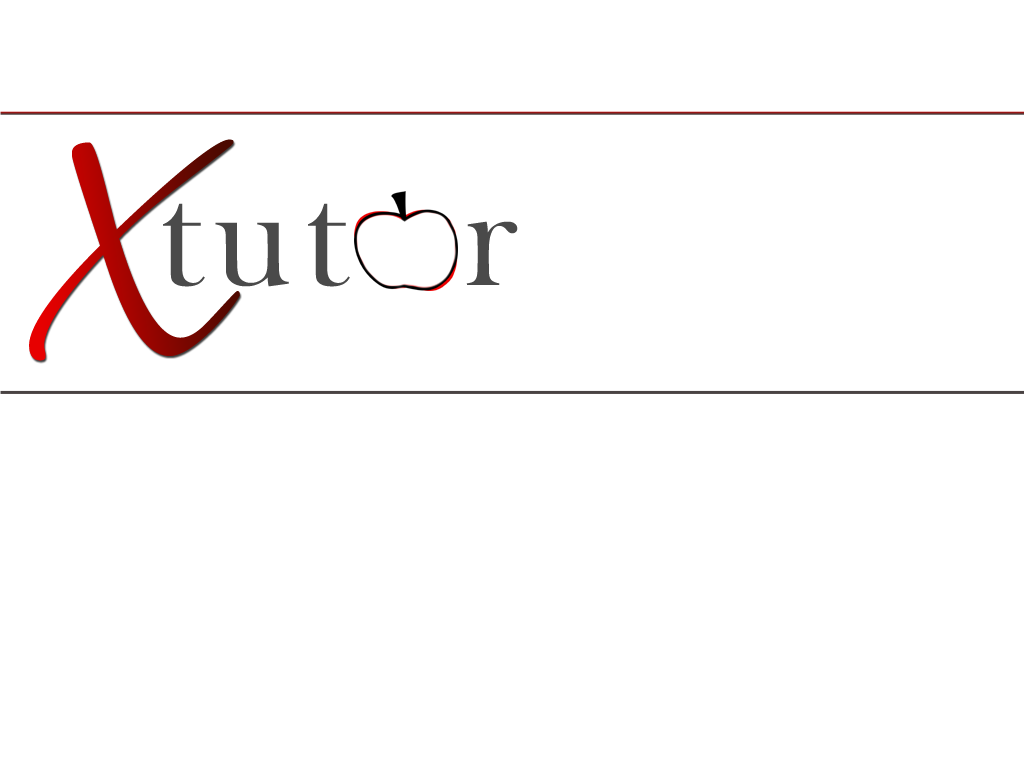Middle School Math6th Grade: Place values Ordering and comparing numbers Patterns Fractions Scientific notation Measurements Ratios Area Volume Geometric figures and calculations 7th Grade: Fractions and decimals (addition, subtraction, multiplication and division) Variables (depictions of relationships through words, symbols, graphs, and tables.) Proportional reasoning symmetry linking geometry and algebra, integers, and probabilities of 2 stage outcomes 8th Grade: Pythagorean theorem Quadratic formula Slope Scatterplots Linear functions and solving systems of linear equations and linear equalities. Other Topics in Middle School Math Algebra: Elementary algebra, basic algebra, pre algebra, algebra 1, algebra 2, intermediate algebra, college algebra, abstract algebra, linear algebra, advanced algebra, matrix algebra, boolean algebra, and discrete math. Geometry: Basic geometry, euclidean geometry, non-euclidean geometry, fractal geometry, analytic geometry, plane geometry, coordinate geometry, solid geometry, algebraic geometry, descriptive geometry, and differential geometry.Problem Solving: Selecting and using appropriate problem solving strategy through logic and proportional reasoning. Calculating distance, rate, time, unit rates and costs via drawings and diagrams (?) Integers: Add, subtract, multiply, and divide integers with like and unlike signs.